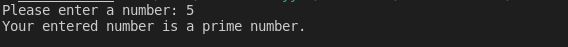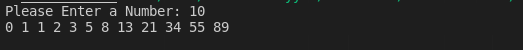Write a c++ program that takes a number as an input and check whether the number entered by the user is prime or composite using for loop and if-else statement.

Sample Program Input

Output

Your entered number is a prime number.

#include <iostream> //Preprogressive Directive
using namespace std; // Global Identifier

int main() // Main Function
{
int number;
cout << "Please enter a number: ";
cin >> number;
bool isPrime = true;

if (number == 1 || number == 0)
{
isPrime = false;
}
else
{
for (int i = 2; i <= number / 2; ++i)
{
if (number % i == 0)
{
isPrime = false;
break;
}
}
}
if (isPrime)
{
cout<<"Your entered number is a prime number.";
}
else
{
cout<<"Your entered number is not a prime number.";
}

return 0;
}c++ programming exercise with solution

Fibonacci Upto n

#include <iostream> //Preprogressive Directive
using namespace std; // Global Identifier

int main() // Main Function
{
int initialNumber = 0, nextNumber = 1, userNumber;c++ programming exercise with solution